# Lesson 2. Open, Plot and Explore Raster Data with Python and Xarray

## Learning Objectives

• Open, plot, and explore raster data using Python.
• Handle no data values in raster data.
• Create plotting extents so you can plot raster and vector data together using matplotlib.
• Explore raster data using histograms and descriptive statistics.

## Open Raster Data in Open Source Python

Remember from the previous lesson that raster or “gridded” data are stored as a grid of values which are rendered on a map as pixels. Each pixel value represents an area on the Earth’s surface. A raster file is composed of regular grid of cells, all of which are the same size. Raster data can be used to store many different types of scientific data including

• elevation data
• canopy height models
• surface temperature
• climate model data outputs
• landuse / landcover data
• and more.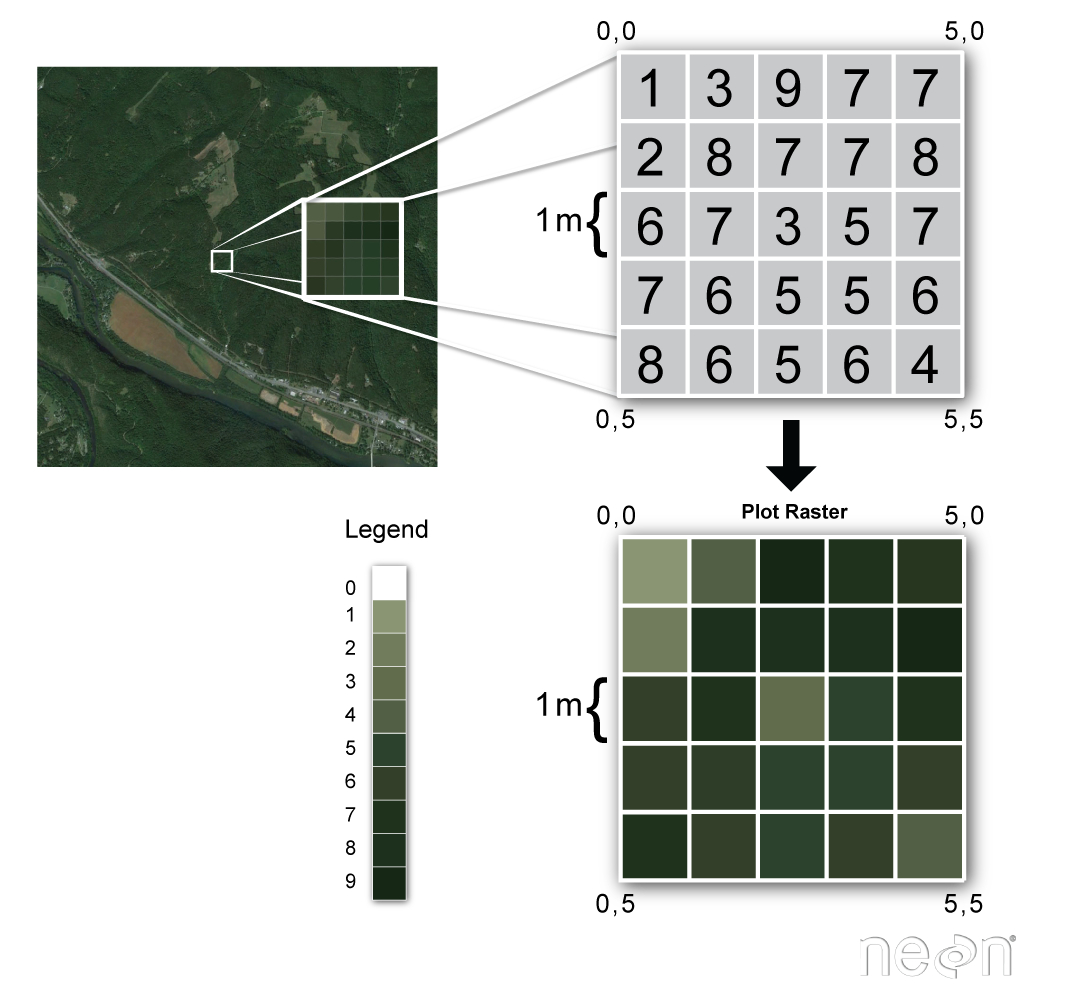A raster is composed of a regular grid of cells. Each cell is the same size in the x and y direction. Source: Colin Williams, NEON.

In this lesson you will learn more about working with lidar derived raster data that represents both terrain / elevation data (elevation of the earth’s surface), and surface elevation (elevation at the tops of trees, buildings etc above the earth’s surface). If you want to read more about how lidar data are used to derive raster based surface models, you can check out this chapter on lidar remote sensing data and the various raster data products derived from lidar data.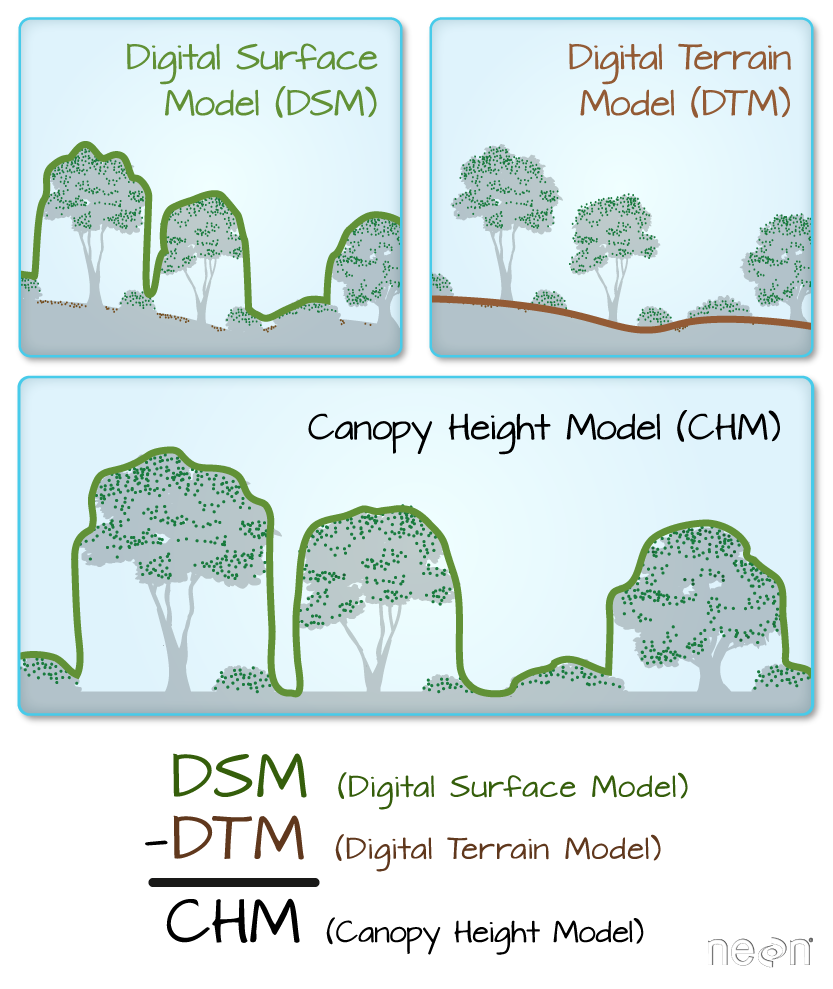Digital Surface Model (DSM), Digital Elevation Models (DEM) and the Canopy Height Model (CHM) are the most common raster format lidar derived data products. One way to derive a CHM is to take the difference between the digital surface model (DSM, tops of trees, buildings and other objects) and the Digital Terrain Model (DTM, ground level). The CHM represents the actual height of trees, buildings, etc. with the influence of ground elevation removed. Graphic: Colin Williams, NEON

Data Tip: The data used in this lesson are NEON (National Ecological Observatory Network) data.

To begin load the packages that you need to process your raster data.

# Import necessary packages
import os

import numpy as np
import matplotlib.pyplot as plt
import seaborn as sns
# Use geopandas for vector data and xarray for raster data
import geopandas as gpd
import rioxarray as rxr

import earthpy as et

# Prettier plotting with seaborn
sns.set(font_scale=1.5, style="white")

# Get data and set working directory
os.chdir(os.path.join(et.io.HOME,
'earth-analytics',
'data'))

Downloading from https://ndownloader.figshare.com/files/16371473


Below, you define the path to a lidar derived digital elevation model (DEM) that was created using NEON (the National Ecological Observatory Network) data.

Data Tip: DEM’s are also sometimes referred to as DTM (Digital Terrain Model or DTM). You can learn more about the 3 lidar derived elevation data types: DEMs, Canopy Height Models (CHM) and Digital Surface Models (DSMs) in the lidar chapter of this textbook.

You then open the data using rioxarray - rxr.open_rasterio("path-to-raster-here").

# Define relative path to file
"spatial",
"boulder-leehill-rd",
"pre-flood",
"lidar",
"pre_DTM.tif")

dtm_pre_arr = rxr.open_rasterio(dem_pre_path)
dtm_pre_arr

<xarray.DataArray (band: 1, y: 2000, x: 4000)>
[8000000 values with dtype=float32]
Coordinates:
* band         (band) int64 1
* y            (y) float64 4.436e+06 4.436e+06 ... 4.434e+06 4.434e+06
* x            (x) float64 4.72e+05 4.72e+05 4.72e+05 ... 4.76e+05 4.76e+05
spatial_ref  int64 0
Attributes:
_FillValue:    -3.4028234663852886e+38
scale_factor:  1.0
grid_mapping:  spatial_ref

When you open raster data using xarray or rioxarray you are creating an xarray.DataArray. The. DataArray object stores the:

• raster data in a numpy array format
• spatial metadata including the CRS, spatial extent of the object

Xarray and numpy provide an efficient way to work with and process raster data. xarray also supports dask and parallel processing which allows you to more efficiently process larger datasets using the processing power that you have on your computer

When you add rioxarray to your package imports, you further get access to spatial data processing using xarray objects. Below, you can view the spatial extent (bounds()) and CRS of the data that you just opened above.

# View the Coordinate Reference System (CRS) & spatial extent
print("The CRS for this data is:", dtm_pre_arr.rio.crs)
print("The spatial extent is:", dtm_pre_arr.rio.bounds())

The CRS for this data is: EPSG:32613
The spatial extent is: (472000.0, 4434000.0, 476000.0, 4436000.0)


The nodata value (or fill value) is also stored in the xarray object.

# View no data value
print("The no data value is:", dtm_pre_arr.rio.nodata)

The no data value is: -3.4028235e+38


Once you have imported your data, you can plot is using xarray.plot().

dtm_pre_arr.plot()
plt.show()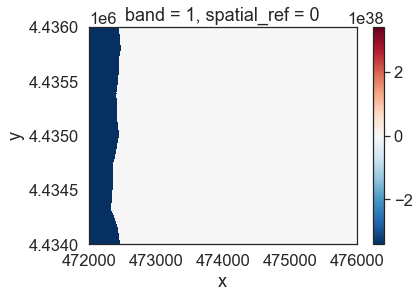When a plot looks off, it is always a good idea to explore whether nodatavalues exist in your data. Often no data values are very large of negative numbers that are not likely to be valid values in your data. These values will skew any plots (or calculations) in your analysis.

The data above should represent terrain model data. However, the range of values is not what is expected. These data are for Boulder, Colorado where the elevation may range from 1000-3000m. There may be some outlier values in the data that may need to be addressed. Below you look at the distribution of pixel values in the data by plotting a histogram.

Notice that there seem to be a lot of pixel values in the negative range in that plot.

# A histogram can also be helpful to look at the range of values in your data
# What do you notice about the histogram below?
dtm_pre_arr.plot.hist(color="purple")
plt.show()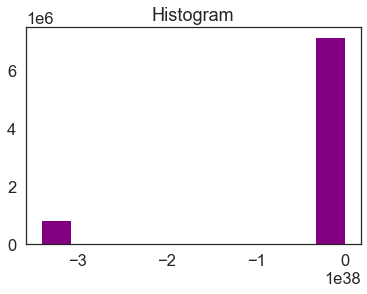Histogram for your lidar DTM. Notice the number of values that are below 0. This suggests that there may be no data values in the data.

Looking at the min and max values of the data, you can see a very small negative number for the minimum. This number matches the nodata value that you looked at above.

print("the minimum raster value is: ", np.nanmin(dtm_pre_arr.values))
print("the maximum raster value is: ", np.nanmax(dtm_pre_arr.values))

the minimum raster value is:  -3.4028235e+38
the maximum raster value is:  2087.43


## Raster Data Exploration - Min and Max Values

Looking at the minimum value of the data, there is one of two things going on that need to be fixed:

1. there may be no data values in the data with a negative value that are skewing your plot colors
2. there also could be outlier data in your raster

You can explore the first option - that there are no data values by reading in the data and masking no data values using the masked=True parameter like this:

rxr.open_rasterio(dem_pre_path, masked=True)

Above you may have also noticed that the array has an additional dimension for the “band”. While the raster only has one layer - there is a 1 in the output of shape that could get in the way of processing.

You can remove that additional dimension using .squeeze()

# Notice that the shape of this object has a 1 at the beginning
# This can cause issues with plotting
dtm_pre_arr.shape

(1, 2000, 4000)

# Open the data and mask no data values
# Squeeze reduces the third dimension given there is only one "band" or layer to this data
# Notice there are now only 2 dimensions to your array
dtm_pre_arr.shape

(2000, 4000)


Plot the data again to see what has changed. Now you have a reasonable range of data values and the data plot as you might expect it to.

# Plot the data and notice that the scale bar looks better
# No data values are now masked
f, ax = plt.subplots(figsize=(10, 5))
dtm_pre_arr.plot(cmap="Greys_r",
ax=ax)
ax.set_title("Lidar Digital Elevation Model (DEM) \n Boulder Flood 2013")
ax.set_axis_off()
plt.show()


The histogram has also changed. Now, it shows a reasonable distribution of pixel values.

f, ax = plt.subplots(figsize=(10, 6))
dtm_pre_arr.plot.hist(color="purple",
bins=20)
ax.set_title("Histogram of the Data with No Data Values Removed")
plt.show()


Notice that now the minimum value looks more like an elevation value (which should most often not be negative).

print("The minimum raster value is: ", np.nanmin(dtm_pre_arr.data))
print("The maximum raster value is: ", np.nanmax(dtm_pre_arr.data))

The minimum raster value is:  1676.2099609375
The maximum raster value is:  2087.429931640625


## Plot Raster and Vector Data Together

If you want, you can also add shapefile overlays to your raster data plot. Below you open a single shapefile using Geopandas that contains a boundary layer that you can overlay on top of your raster dataset. You will learn more about working with shapefiles in Geopandas in this chapter of the earthdatascience.org intermediate textbook.

# Open site boundary vector layer
"spatial",
"boulder-leehill-rd",
"clip-extent.shp")

# Plot the vector data
f, ax = plt.subplots(figsize=(8,4))
site_bound_shp.plot(color='teal',
edgecolor='black',
ax=ax)
ax.set(title="Site Boundary Layer - Shapefile")
plt.show()


Once you have your shapefile open, can plot the two datasets together and begin to create a map.

f, ax = plt.subplots(figsize=(11, 4))

dtm_pre_arr.plot.imshow(cmap="Greys",
ax=ax)
site_bound_shp.plot(color='None',
edgecolor='teal',
linewidth=2,
ax=ax,
zorder=4)

ax.set(title="Raster Layer with Vector Overlay")
ax.axis('off')
plt.show()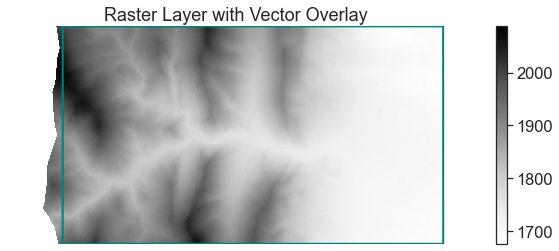DTM raster layer with a vector study area extent overlayed on top.

Data Tip: Customizing Raster Plot Color Ramps To change the color of a raster plot you set the colormap. Matplotlib has a list of pre-determined color ramps that you can chose from. You can reverse a color ramp by adding _r at the end of the color ramp’s name, for example cmap = 'viridis' vs cmap = 'viridis_r'.

You now have the basic skills needed to open and plot raster data using rioxarray and xarray. In the following lessons, you will learn more about exploring and processing raster data.